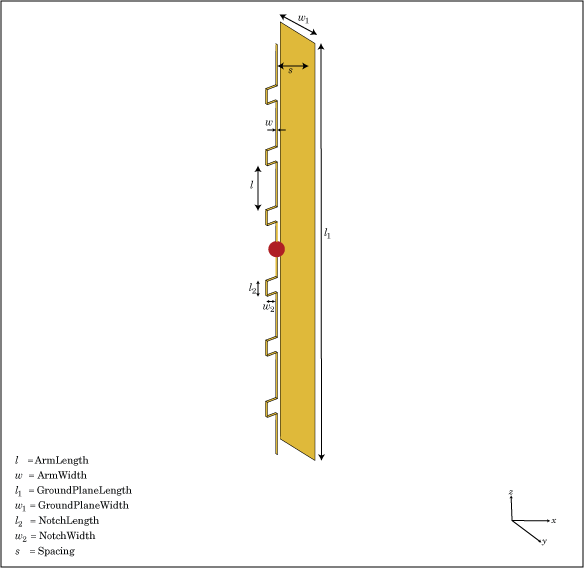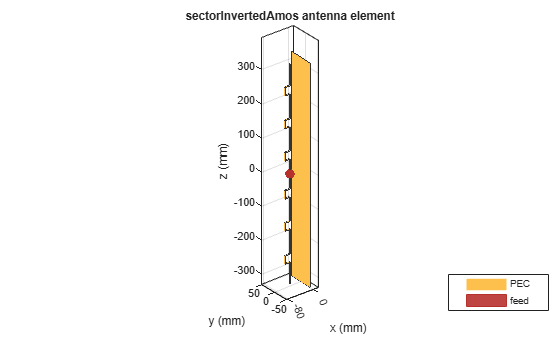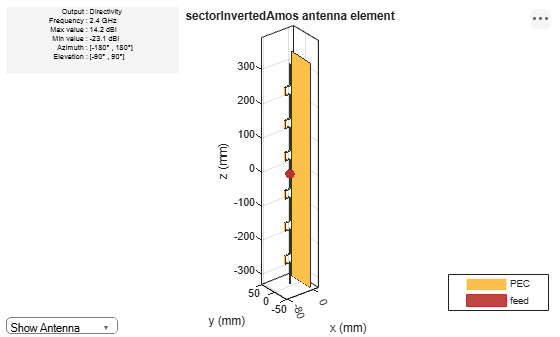# sectorInvertedAmos

Create inverted Amos sector antenna

## Description

Use the `sectorInvertedAmos` object to create an inverted Amos sector antenna consisting of four dipole-like arms. The antenna is fed at the origin of the dipole. The dipole arms are symmetric about the origin. The operating frequency of the antenna is at 2.45 GHz wireless.## Creation

### Syntax

``amossector = sectorInvertedAmos``
``amossector = sectorInvertedAmos(Name,Value)``

### Description

example

````amossector = sectorInvertedAmos` creates an inverted Amos sector antenna with four dipole-like arms.```
````amossector = sectorInvertedAmos(Name,Value)` sets properties using one or more name-value pair. For example, `amossector = sectorInvertedAmos('ArmWidth',0.2)` creates an inverted Amos sector with a dipole width of 0.2 m. Enclose each property name in quotes.```

## Properties

expand all

Length of individual dipole arms, specified as a vector with each element unit in meters.

Example: ```'ArmLength',[0.0980 0.0810 0.0830 0.0750]```

Example: ```amossector.ArmLength = [0.0980 0.0810 0.0830 0.0750]```

Data Types: `double`

Width of dipole arms, specified as a scalar in meters.

Example: `'ArmWidth',0.0025`

Example: `amossector.ArmWidth = 0.0025`

Data Types: `double`

Notch length, specified as a scalar in meters. For an inverted Amos sector antenna with seven stacked arms, six notches are generated. Notch length is measured along the length of the antennas.

Example: `'NotchLength',0.001`

Example: `amossector.NotchLength = 0.001`

Data Types: `double`

Notch width, specified as a scalar in meters. For an inverted Amos sector antenna with seven stacked arms, six notches are generated. Notch width is measured perpendicular to the length of the antenna.

Example: `'NotchWidth',0.00190`

Example: `amossector.NotchWidth = 0.00190`

Data Types: `double`

Ground plane length, specified as a scalar in meters. By default, ground plane length is measured along x-axis.

Example: `'GroundPlaneLength',0.7500`

Example: ```amossector.GroundPlaneLength = 0.7500```

Data Types: `double`

Ground plane width, specified as a scalar in meters. By default, ground plane width is measured along y-axis.

Example: `'GroundPlaneWidth',0.0500`

Example: ```amossector.GroundPlaneWidth = 0.0500```

Data Types: `double`

Distance between ground plane and antenna element, specified as a scalar in meters.

Example: `'Spacing',0.0355`

Example: `amossector.Spacing = 0.0355`

Data Types: `double`

Type of the metal used as a conductor, specified as a metal material object. You can choose any metal from the `MetalCatalog` or specify a metal of your choice. For more information, see `metal`. For more information on metal conductor meshing, see Meshing.

Example: ```m = metal('Copper'); 'Conductor',m```

Example: ```m = metal('Copper'); ant.Conductor = m```

Lumped elements added to the antenna feed, specified as a lumped element object. You can add a load anywhere on the surface of the antenna. By default, it is at the origin. For more information, see `lumpedElement`.

Example: `'Load',lumpedelement`. `lumpedelement` is the object for the load created using `lumpedElement`.

Example: ```amossector.Load = lumpedElement('Impedance',75)```

Tilt angle of the antenna, specified as a scalar or vector with each element unit in degrees. For more information, see Rotate Antennas and Arrays.

Example: `Tilt=90`

Example: `Tilt=[90 90]`,`TiltAxis=[0 1 0;0 1 1]` tilts the antenna at 90 degrees about the two axes defined by the vectors.

Note

The `wireStack` antenna object only accepts the dot method to change its properties.

Data Types: `double`

Tilt axis of the antenna, specified as:

• Three-element vector of Cartesian coordinates in meters. In this case, each coordinate in the vector starts at the origin and lies along the specified points on the X-, Y-, and Z-axes.

• Two points in space, each specified as three-element vectors of Cartesian coordinates. In this case, the antenna rotates around the line joining the two points in space.

• A string input describing simple rotations around one of the principal axes, 'X', 'Y', or 'Z'.

Example: `TiltAxis=[0 1 0]`

Example: `TiltAxis=[0 0 0;0 1 0]`

Example: `TiltAxis = 'Z'`

Data Types: `double`

## Object Functions

 `show` Display antenna, array structures or shapes `axialRatio` Axial ratio of antenna `beamwidth` Beamwidth of antenna `charge` Charge distribution on antenna or array surface `current` Current distribution on antenna or array surface `design` Design prototype antenna or arrays for resonance around specified frequency `efficiency` Radiation efficiency of antenna `EHfields` Electric and magnetic fields of antennas; Embedded electric and magnetic fields of antenna element in arrays `impedance` Input impedance of antenna; scan impedance of array `mesh` Mesh properties of metal, dielectric antenna, or array structure `meshconfig` Change mesh mode of antenna structure `optimize` Optimize antenna or array using SADEA optimizer `pattern` Radiation pattern and phase of antenna or array; Embedded pattern of antenna element in array `patternAzimuth` Azimuth pattern of antenna or array `patternElevation` Elevation pattern of antenna or array `rcs` Calculate and plot radar cross section (RCS) of platform, antenna, or array `returnLoss` Return loss of antenna; scan return loss of array `sparameters` Calculate S-parameter for antenna and antenna array objects `vswr` Voltage standing wave ratio of antenna

## Examples

collapse all

Create and view an inverted Amos sector antenna.

`sectoria = sectorInvertedAmos`
```sectoria = sectorInvertedAmos with properties: ArmLength: [0.0880 0.0710 0.0730 0.0650] ArmWidth: 0.0040 NotchLength: 0.0238 NotchWidth: 0.0170 GroundPlaneLength: 0.6600 GroundPlaneWidth: 0.0750 Spacing: 0.0355 Conductor: [1x1 metal] Tilt: 0 TiltAxis: [1 0 0] Load: [1x1 lumpedElement] ```
`show(sectoria)`Plot Radiation Pattern at 2.4 GHz

`pattern(sectoria,2.4e9)`## Version History

Introduced in R2017b